# Commutative group scheme

(diff) ← Older revision | Latest revision (diff) | Newer revision → (diff)
Jump to: navigation, search

A group schemeover a basis scheme, the value of which on any-scheme is an Abelian group. Examples of commutative group schemes are Abelian schemes and algebraic tori (cf. Algebraic torus; Abelian scheme). A generalization of algebraic tori in the framework of the theory of group schemes is the following notion. A commutative group scheme is said to be a group scheme of multiplicative type if for any pointthere is an open neighbourhood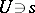and and an absolutely-flat quasi-compact morphism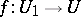such that the commutative group schemeis diagonalizable over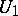. Here, a diagonalizable group scheme is a group scheme of the form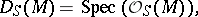whereis an Abelian group and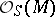is its group algebra with coefficients in the structure sheafof the scheme. In the case whenis the spectrum of an algebraically closed field, this notion reduces to that of a diagonalizable group. If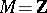is the additive group of integers, then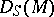coincides with the multiplicative group scheme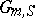.

Letbe a group scheme overwhose fibre over the point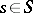is a group scheme of multiplicative type over the residue class field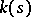. Then there is a neighbourhoodofsuch that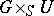is a group scheme of multiplicative type over(Grothendieck's rigidity theorem).

The structure of commutative group schemes has been studied in the case when the basis schemeis the spectrum of a field, and the commutative group schemeis of finite type over. In this case the commutative group scheme contains a maximal invariant affine group subscheme, the quotient with respect to which is an Abelian variety (a structure theorem of Chevalley). Any affine commutative group schemeof such a type has a maximal invariant group subscheme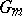of multiplicative type, the quotient with respect to which is a unipotent group. If the fieldis perfect, then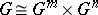, where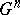is a maximal unipotent subgroup of.

How to Cite This Entry:
Commutative group scheme. Encyclopedia of Mathematics. URL: http://encyclopediaofmath.org/index.php?title=Commutative_group_scheme&oldid=13667
This article was adapted from an original article by I.V. Dolgachev (originator), which appeared in Encyclopedia of Mathematics - ISBN 1402006098. See original article